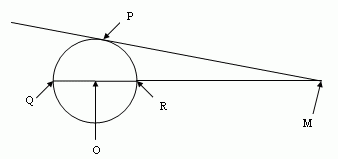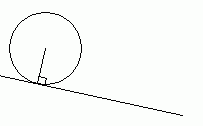SEARCH HOMEMath Central Quandaries & QueriesQuestion from Sean, a student: Let M be a point outside a circle, and let a line through M be tangent to the circle at point P.  Let the line through M and the center of the circle intersect the circle in points Q, R. Prove that │PM│2  = │MQ│ x │MR│Hi Sean.

The key is this property of circle geometry:

The angle formed by the tangent to a circle and the radius to the point of tangency is a right angle.Draw line OP and label your lengths, recognizing that |OQ| = |OR| = |OP| = radius. Then use the Pythagorean Theorem to complete your question with simple algebra.

Hope this helps,
Stephen La Rocque.Math Central is supported by the University of Regina and The Pacific Institute for the Mathematical Sciences.